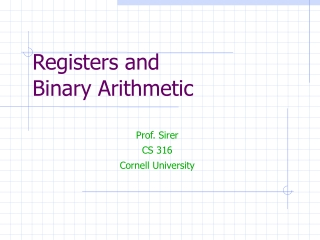Download PresentationRegisters and Binary Arithmetic

# Registers and Binary Arithmetic - PowerPoint PPT PresentationDownload Presentation## Registers and Binary Arithmetic

- - - - - - - - - - - - - - - - - - - - - - - - - - - E N D - - - - - - - - - - - - - - - - - - - - - - - - - - -
##### Presentation Transcript

1. Prof. Sirer CS 316 Cornell University Registers andBinary Arithmetic

2. Recap • We can build combinatorial circuits • Gates, Karnaugh maps, minimization • We can build stateful circuits • Record 1-bit values in latches and flip-flops • Powerful combination • We can build real, useful devices • But we will often need to perform arithmetic

3. Binary Arithmetic 12 + 25 37 001100 + 011010 100110 • Arithmetic works the same way regardless of base • Add the digits in each position • Propagate the carry • Unsigned binary addition is pretty easy • Combine two bits at a time • Along with a carry

4. 1-bit Adder A0 B0 Cout R0 • Adds two 1-bit numbers, computes 1-bit result and carry out • Useful for the rightmost binary digit, not much else

5. 1-bit Adder with Carry Ai Bi Cout Cin Ri • Adds two 1-bit numbers, along with carry-in, computes 1-bit result and carry out • Can be cascaded to add N-bit numbers

6. 4-bit Adder A3 B3 A2 B2 A1 B1 A0 B0 Overflow 0 R3 R2 R1 R0 • Adds two 4-bit numbers, along with carry-in, computes 4-bit result and overflow • Overflow indicates that the result does not fit in 4 bits

7. Arithmetic with Negative Numbers • Negative numbers complicate arithmetic • Recall that for addition and subtraction, the rules are: • Both positive => add, result positive • One +, one - => subtract small number from larger one • Both negative => add, result negative • We could represent sign with an explicit bit • the “sign-magnitude form” • But arithmetic would be much easier to perform in hardware if we did not have to examine the operands’ signs • Two’s complement representation enables arithmetic to be performed without examining the operands

8. Two’s Complement • Nonnegative numbers are represented as usual • 0 = 0000 • 1 = 0001 • 3 = 0011 • 7 = 0111 • To negate a number, flip all bits, add one • -1: 1  0001  1110  1111 • -3: 3  0011  1100  1101 • -7: 7  0111  1000  1001 • -0: 0  0000  1111  0000 (this is good, -0 = +0)

9. Two’s Complement Addition • Perform addition as usual, regardless of sign • 1 = 0001, 3 = 0011, 7 = 0111, 0 = 0000 • -1 = 1111, -3 = 1101, -7 = 1001 • Examples • 1 + -1 = 1111 + 0001 = 0000 (0) • -3 + -1 = 1111 + 1101 = 1100 (-4) • -7 + 3 = 1001 + 0011 = 1100 (-4)

10. Two’s Complement Facts • Negative numbers have a leading 1 • Similar to signed magnitude form • Largest negative=1000…0; positive=0111…1 • N bits can be used to represent • unsigned: the range 0..2N-1 • ex: 8 bits  0..255 • two’s complement: the range –(2N-1).. (2N-1)-1 • ex: 8 bits  (10000000)..(01111111)  -128..127 • Overflow • carry into most significant bit (msb) != carry out of msb

11. Two’s Complement Adder • Let’s build a two’s complement adder • Already built, just needed to modify overflow checking A3 B3 A2 B2 A1 B1 A0 B0 overflow 0 R3 R2 R1 R0

12. Two’s Complement Subtraction • Subtraction is simply addition, where one of the operands has been negated • Negation is done by inverting all bits and adding one B3 B2 B1 B0 A3 A2 A1 A0 1 R3 R2 R1 R0

13. A Calculator • User enters the numbers to be added or subtracted using toggle switches • User selects ADD or SUBTRACT • Muxes feed A and B,or A and –B, to the 8-bit adder • The 8-bit decoder for the hex display is straightforward (but not shown in detail) 8 … reg 8 led-dec adder 8 8 … mux reg 8 0 1 mux add/sub select doit

14. Summary • We can now perform arithmetic • And build basic circuits that operate on numbers